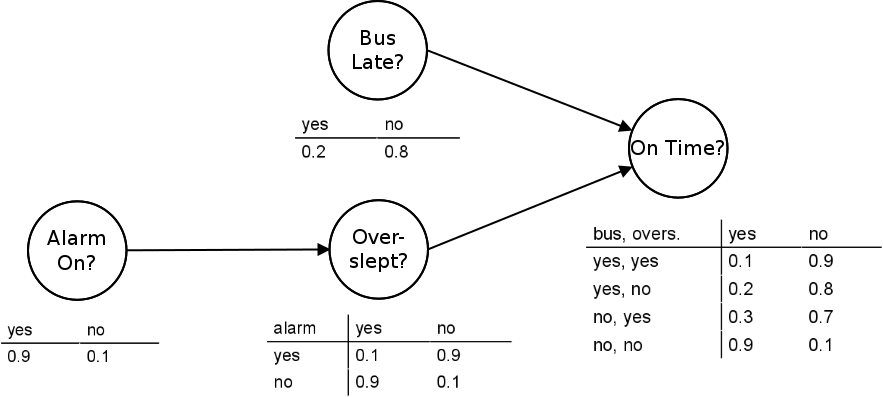Bayesian networks

# Bayesian networks

We study structure learning in Bayesian networks.Bayesian network
Photo:
Pekka Parviainen

Bayesian networks are a widely-used class of probabilistic graphical models. They consist of two parts: a structure and parameters. The structure is a directed acyclic graph (DAG) that expresses conditional independencies and dependencies among ran- dom variables associated with nodes. The parameters consist of conditional probability distributions associated with each node. A Bayesian network is a compact, flexible and interpretable representation of a joint probability distribution. It is also an useful tool in knowledge discovery as directed acyclic graphs allow representing causal relations between variables. Typically, a Bayesian network is learned from data.

We study Bayesian networks, especially learning their structure. We are interested in scalable algorithms with theoretical guarantees.